# Recipes¶

## FOX.recipes.param¶

A set of functions for analyzing and plotting ARMC results.

Examples

A general overview of the functions within this module.

>>> import pandas as pd
>>> from FOX.recipes import get_best, overlay_descriptor, plot_descriptor

>>> hdf5_file: str = ...

>>> param: pd.Series = get_best(hdf5_file, name='param')  # Extract the best parameters
>>> rdf: pd.DataFrame = get_best(hdf5_file, name='rdf')  # Extract the matching RDF

# Compare the RDF to its reference RDF and plot
>>> rdf_dict = overlay_descriptor(hdf5_file, name='rdf')
>>> plot_descriptor(rdf_dict)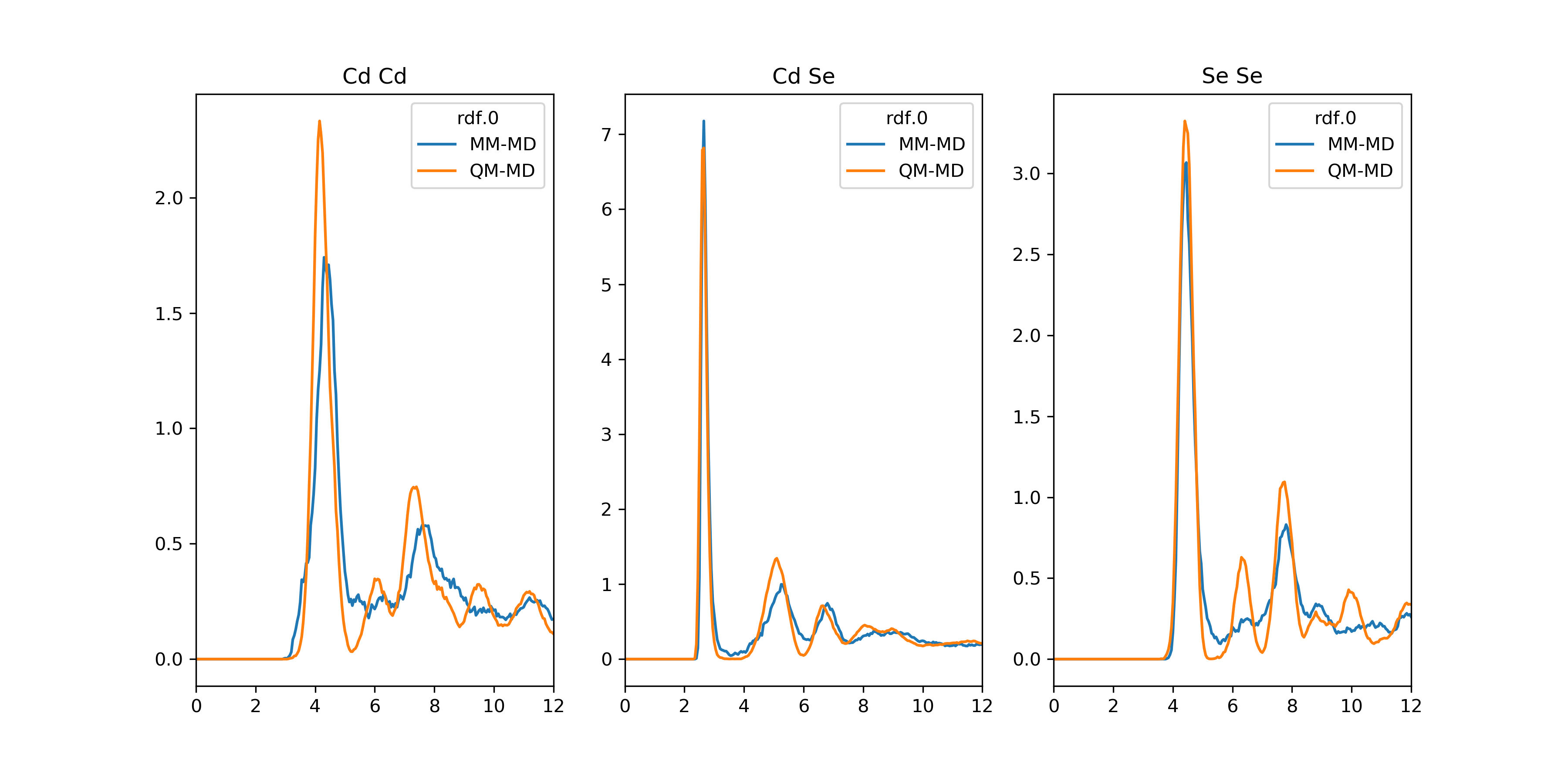Examples

A small workflow for calculating for calculating free energies using distribution functions such as the radial distribution function (RDF).

>>> import pandas as pd
>>> from FOX import get_free_energy
>>> from FOX.recipes import get_best, overlay_descriptor, plot_descriptor

>>> hdf5_file: str = ...

>>> rdf: pd.DataFrame = get_best(hdf5_file, name='rdf')
>>> G: pd.DataFrame = get_free_energy(rdf, unit='kcal/mol')

>>> rdf_dict = overlay_descriptor(hdf5_file, name='rdf)
>>> G_dict = {key: get_free_energy(value) for key, value in rdf_dict.items()}
>>> plot_descriptor(G_dict)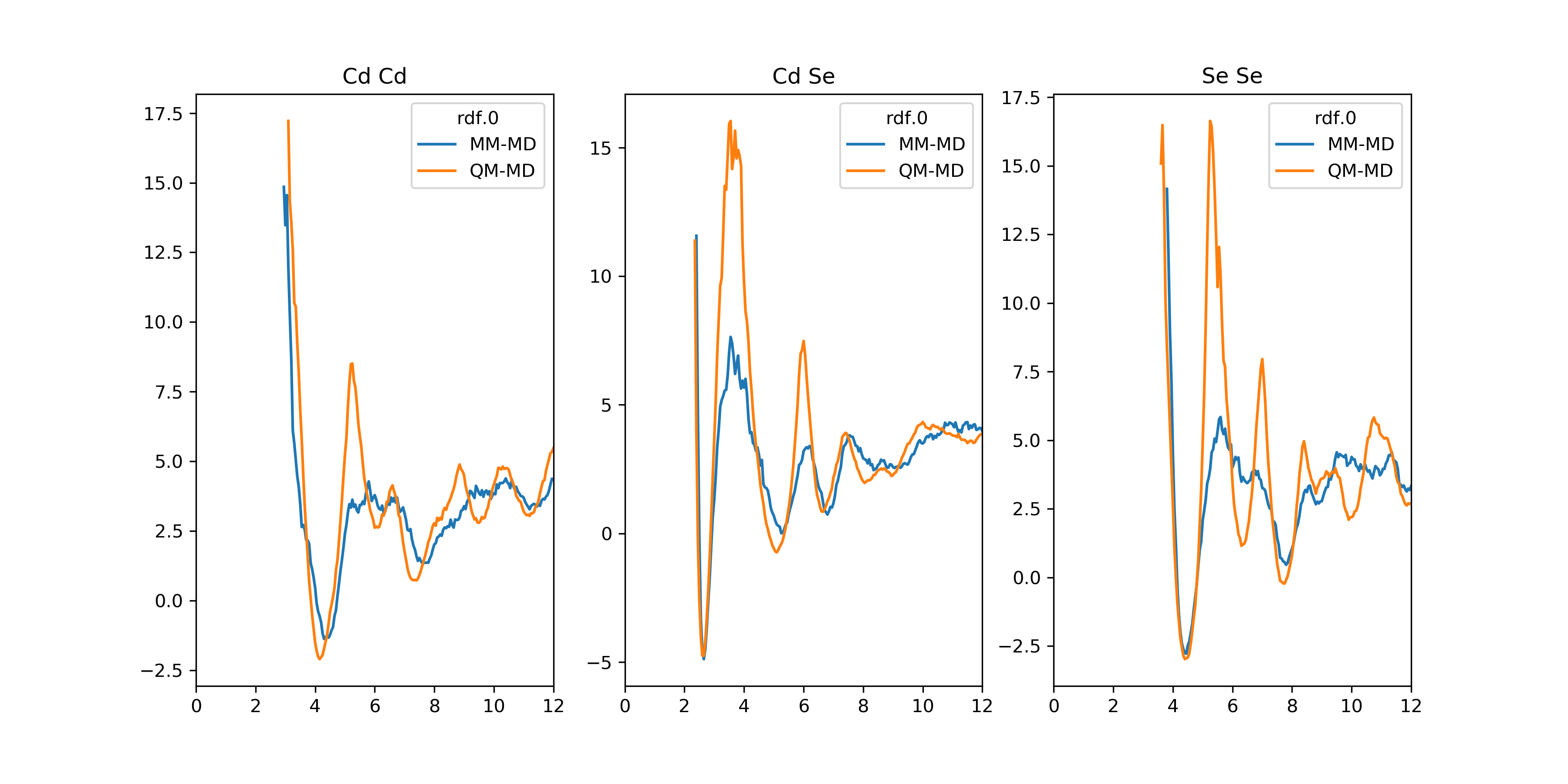Examples

A workflow for plotting parameters as a function of ARMC iterations.

>>> import numpy as np
>>> import pandas as pd
>>> from FOX import from_hdf5
>>> from FOX.recipes import plot_descriptor

>>> hdf5_file: str = ...

>>> param: pd.DataFrame = from_hdf5(hdf5_file, 'param')
>>> param.index.name = 'ARMC iteration'
>>> param_dict = {key: param[key] for key in param.columns.levels}

>>> plot_descriptor(param_dict)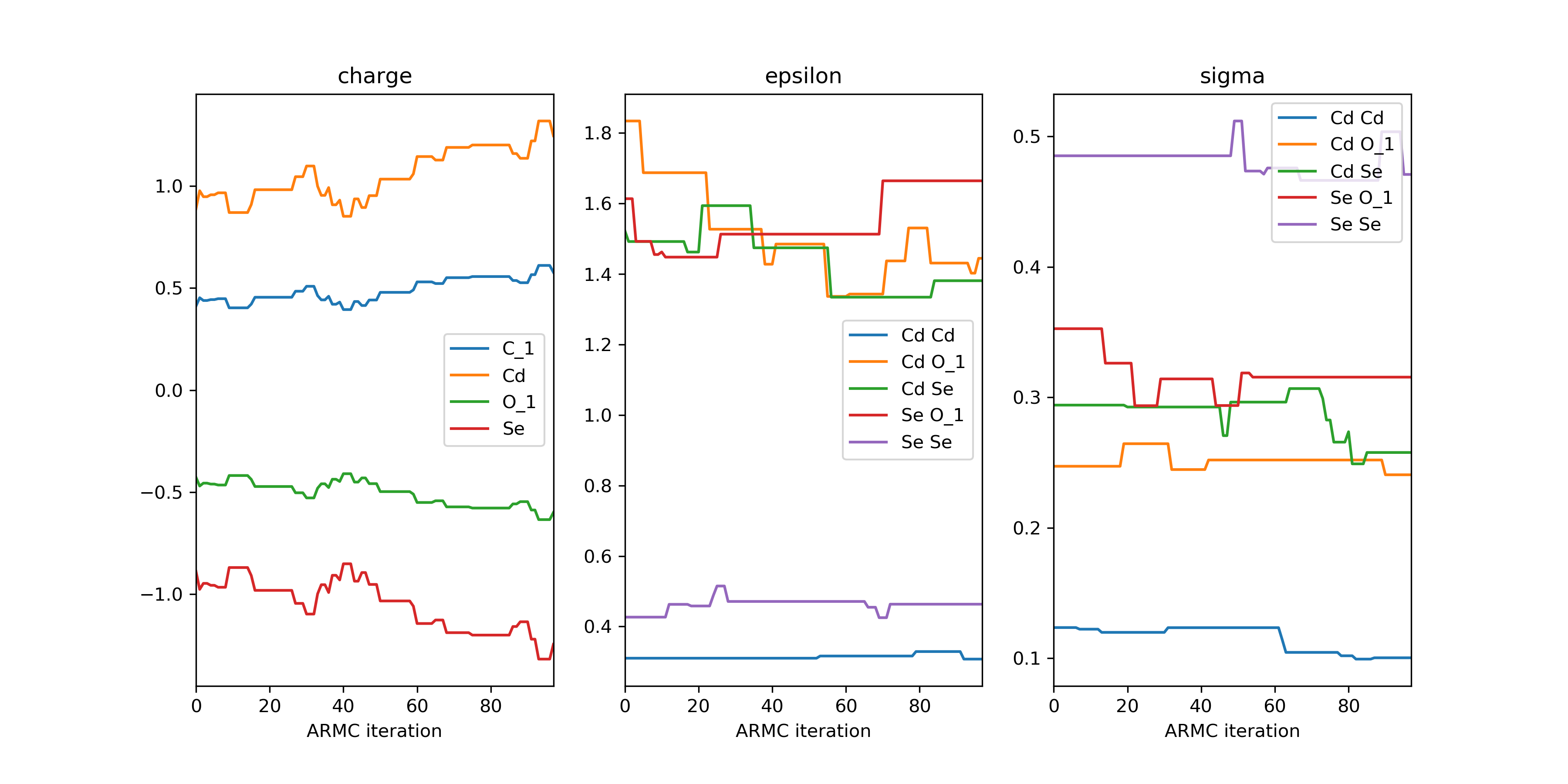This approach can also be used for the plotting of other properties such as the auxiliary error.

>>> ...

>>> err: pd.DataFrame = from_hdf5(hdf5_file, 'aux_error')
>>> err.index.name = 'ARMC iteration'
>>> err_dict = {'Auxiliary Error': err}

>>> plot_descriptor(err_dict)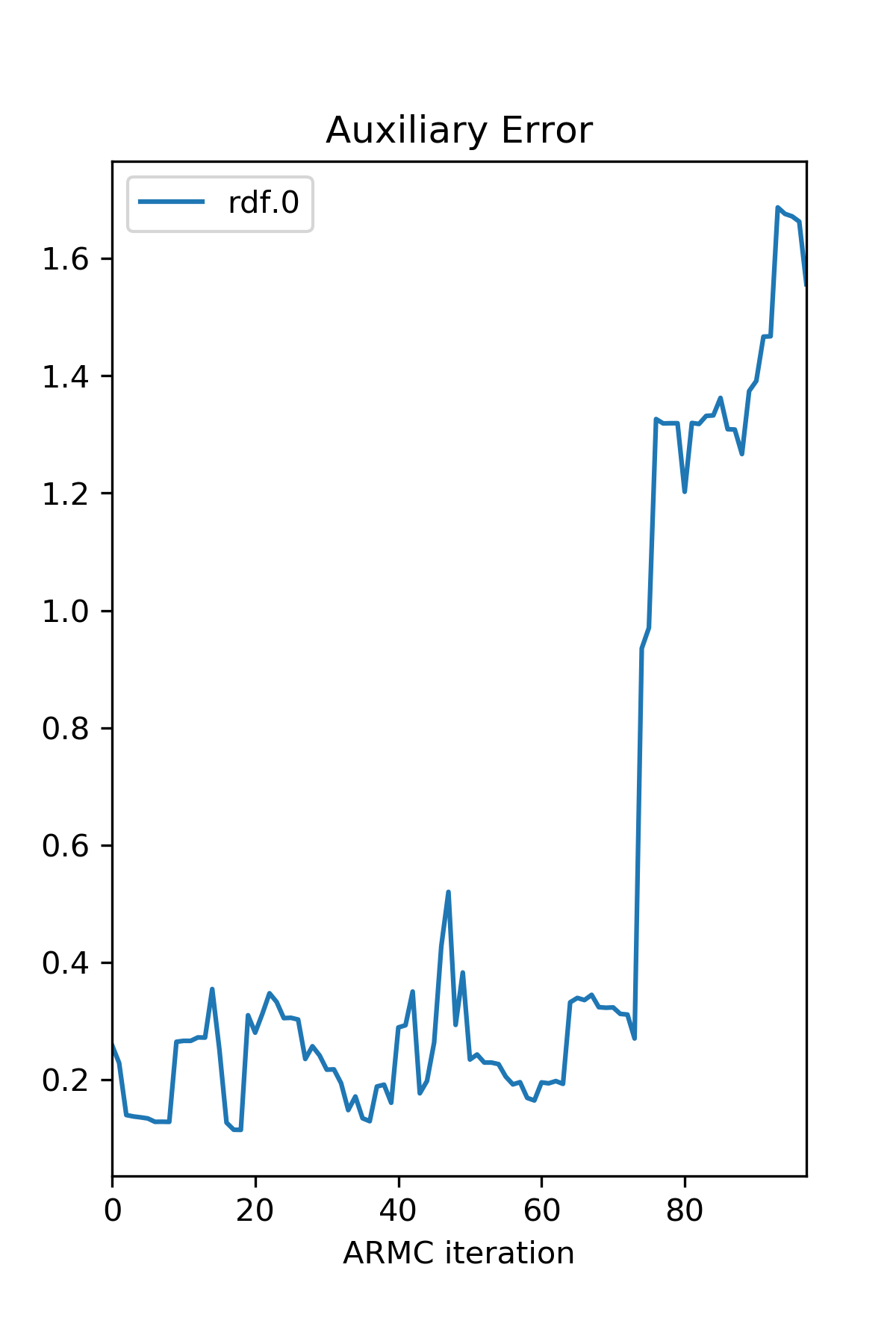On occasion it might be desirable to only print the error of, for example, accepted iterations. Given a sequence of booleans (bool_seq), one can slice a DataFrame or Series (df) using df.loc[bool_seq].

>>> ...

>>> acceptance: np.ndarray = from_hdf5(hdf5_file, 'acceptance')  # Boolean array
>>> err_slice_dict = {key: df.loc[acceptance], value for key, df in err_dict.items()}

>>> plot_descriptor(err_slice_dict)


### Index¶

 get_best(hdf5_file[, name, i]) Return the PES descriptor or ARMC property which yields the lowest error. overlay_descriptor(hdf5_file[, name, i]) Return the PES descriptor which yields the lowest error and overlay it with the reference PES descriptor. plot_descriptor(descriptor[, show_fig, …]) Plot a DataFrame or iterable consisting of one or more DataFrames.

### API¶

FOX.recipes.param.get_best(hdf5_file, name='rdf', i=0)[source]

Return the PES descriptor or ARMC property which yields the lowest error.

Parameters: hdf5_file (str) – The path+filename of the ARMC .hdf5 file. name (str) – The name of the PES descriptor, e.g. "rdf". Alternatively one can supply an ARMC property such as "acceptance", "param" or "aux_error". i (int) – The index of the desired PES. Only relevant for PES-descriptors of state-averaged ARMCs. A DataFrame of the optimal PES descriptor or other (user-specified) ARMC property. pandas.DataFrame or pd.Series
FOX.recipes.param.overlay_descriptor(hdf5_file, name='rdf', i=0)[source]

Return the PES descriptor which yields the lowest error and overlay it with the reference PES descriptor.

Parameters: hdf5_file (str) – The path+filename of the ARMC .hdf5 file. name (str) – The name of the PES descriptor, e.g. "rdf". i (int) – The index of desired PES. Only relevant for state-averaged ARMCs. A dictionary of DataFrames. Values consist of DataFrames with two keys: "MM-MD" and "QM-MD". Atom pairs, such as "Cd Cd", are used as keys.
FOX.recipes.param.plot_descriptor(descriptor, show_fig=True, kind='line', sharex=True, sharey=False, **kwargs)[source]

Plot a DataFrame or iterable consisting of one or more DataFrames.

Requires the matplotlib package.

Parameters: descriptor (pandas.DataFrame or Iterable [pandas.DataFrame]) – A DataFrame or an iterable consisting of DataFrames. show_fig (bool) – Whether to show the figure or not. kind (str) – The plot kind to-be passed to pandas.DataFrame.plot(). sharex/sharey (bool) – Whether or not the to-be created plots should share their x/y-axes. **kwargs (Any) – Further keyword arguments for the pandas.DataFrame.plot() method. A matplotlib Figure. Figure

See also

get_best()
Return the PES descriptor or ARMC property which yields the lowest error.
overlay_descriptor()
Return the PES descriptor which yields the lowest error and overlay it with the reference PES descriptor.

## FOX.recipes.psf¶

A set of functions for creating .psf files.

Examples

Example code for generating a .psf file. Ligand atoms within the ligand .xyz file and the qd .xyz file should be in the exact same order. For example, implicit hydrogen atoms added by the from_smiles functions are not guaranteed to be ordered, even when using canonical SMILES strings.

>>> from scm.plams import Molecule, from_smiles
>>> from FOX import PSFContainer
>>> from FOX.recipes import generate_psf

# Accepts .xyz, .pdb, .mol or .mol2 files
>>> qd = Molecule(...)
>>> ligand: Molecule = Molecule(...)
>>> rtf_file : str = ...
>>> psf_file : str = ...

>>> psf: PSFContainer = generate_psf(qd_xyz, ligand_xyz, rtf_file=rtf_file)
>>> psf.write(psf_file)


Examples

If no ligand .xyz is on hand, or its atoms are in the wrong order, it is possible the extract the ligand directly from the quantum dot. This is demonstrated below with oleate ($$C_{18} H_{33} O_{2}^{-}$$).

>>> from scm.plams import Molecule
>>> from FOX import PSFContainer
>>> from FOX.recipes import generate_psf, extract_ligand

>>> qd = Molecule(...)  # Accepts an .xyz, .pdb, .mol or .mol2 file
>>> rtf_file : str = ...

>>> ligand_len = 18 + 33 + 2
>>> ligand_atoms = {'C', 'H', 'O'}
>>> ligand: Molecule = extract_ligand(qd, ligand_len, ligand_atoms)

>>> psf: PSFContainer = generate_psf(qd, ligand, rtf_file=rtf_file)
>>> psf.write(...)


Examples

Example for multiple ligands.

>>> from typing import List
>>> from scm.plams import Molecule
>>> from FOX import PSFContainer
>>> from FOX.recipes import generate_psf2

>>> qd = Molecule(...)  # Accepts an .xyz, .pdb, .mol or .mol2 file
>>> ligands = ('C[O-]', 'CC[O-]', 'CCC[O-]')
>>> rtf_files = (..., ..., ...)

>>> psf: PSFContainer = generate_psf2(qd, *ligands, rtf_file=rtf_files)
>>> psf.write(...)


If the the psf construction with generate_psf2() failes to identify a particular ligand, it is possible to return all (failed) potential ligands with the ret_failed_lig parameter.

>>> ...

>>> ligands = ('CCCCCCCCC[O-]', 'CCCCBr')
>>> failed_mol_list: List[Molecule] = generate_psf2(qd, *ligands, ret_failed_lig=True)


### Index¶

 generate_psf(qd, ligand[, rtf_file, str_file]) Generate a PSFContainer instance for qd. generate_psf2(qd, *ligands[, rtf_file, …]) Generate a PSFContainer instance for qd with multiple different ligands. extract_ligand(qd, ligand_len, ligand_atoms) Extract a single ligand from qd.

### API¶

FOX.recipes.psf.generate_psf(qd, ligand, rtf_file=None, str_file=None)[source]

Generate a PSFContainer instance for qd.

Parameters: qd (str or Molecule) – The ligand-pacifated quantum dot. Should be supplied as either a Molecule or .xyz file. ligand (str or Molecule) – A single ligand. Should be supplied as either a Molecule or .xyz file. rtf_file (str, optional) – The path+filename of the ligand’s .rtf file. Used for assigning atom types. Alternativelly, one can supply a .str file with the str_file argument. str_file (str, optional) – The path+filename of the ligand’s .str file. Used for assigning atom types. Alternativelly, one can supply a .rtf file with the rtf_file argument. A PSFContainer instance with the new .psf file. PSFContainer
FOX.recipes.psf.generate_psf2(qd, *ligands, rtf_file=None, str_file=None, ret_failed_lig=False)[source]

Generate a PSFContainer instance for qd with multiple different ligands.

Parameters: qd (str or Molecule) – The ligand-pacifated quantum dot. Should be supplied as either a Molecule or .xyz file. *ligands (str, Molecule or Chem.Mol) – One or more PLAMS/RDkit Molecules and/or SMILES strings representing ligands. rtf_file (str or Iterable [str], optional) – The path+filename of the ligand’s .rtf files. Filenames should be supplied in the same order as ligands. Used for assigning atom types. Alternativelly, one can supply a .str file with the str_file argument. str_file (str or Iterable [str], optional) – The path+filename of the ligand’s .str files. Filenames should be supplied in the same order as ligands. Used for assigning atom types. Alternativelly, one can supply a .rtf file with the rtf_file argument. ret_failed_lig (bool) – If True, return a list of all failed (potential) ligands if the function cannot identify any ligands within a certain range. Usefull for debugging. If False, raise a MoleculeError. A single ligand Molecule. Molecule MoleculeError – Raised if the function fails to identify any ligands within a certain range. If ret_failed_lig = True, return a list of failed (potential) ligands instead and issue a warning.
FOX.recipes.psf.extract_ligand(qd, ligand_len, ligand_atoms)[source]

Extract a single ligand from qd.

Parameters: qd (str or Molecule) – The ligand-pacifated quantum dot. Should be supplied as either a Molecule or .xyz file. ligand_len (int) – The number of atoms within a single ligand. ligand_atoms (str or Iterable [str]) – One or multiple strings with the atomic symbols of all atoms within a single ligand. A single ligand Molecule. Molecule

## FOX.recipes.ligands¶

A set of functions for analyzing ligands.

Examples

An example for generating a ligand center of mass RDF.

>>> import numpy as np
>>> import pandas as pd
>>> from FOX import MultiMolecule, example_xyz
>>> from FOX.recipes import get_lig_center

>>> mol = MultiMolecule.from_xyz(example_xyz)
>>> start = 123  # Start of the ligands
>>> step = 4  # Size of the ligands

# Add dummy atoms to the ligand-center of mass and calculate the RDF
>>> lig_centra: np.ndarray = get_lig_center(mol, start, step)
>>> mol_new: MultiMolecule = mol.add_atoms(lig_centra, symbols='Xx')
>>> rdf: pd.DataFrame = mol_new.init_rdf(atom_subset=['Xx'])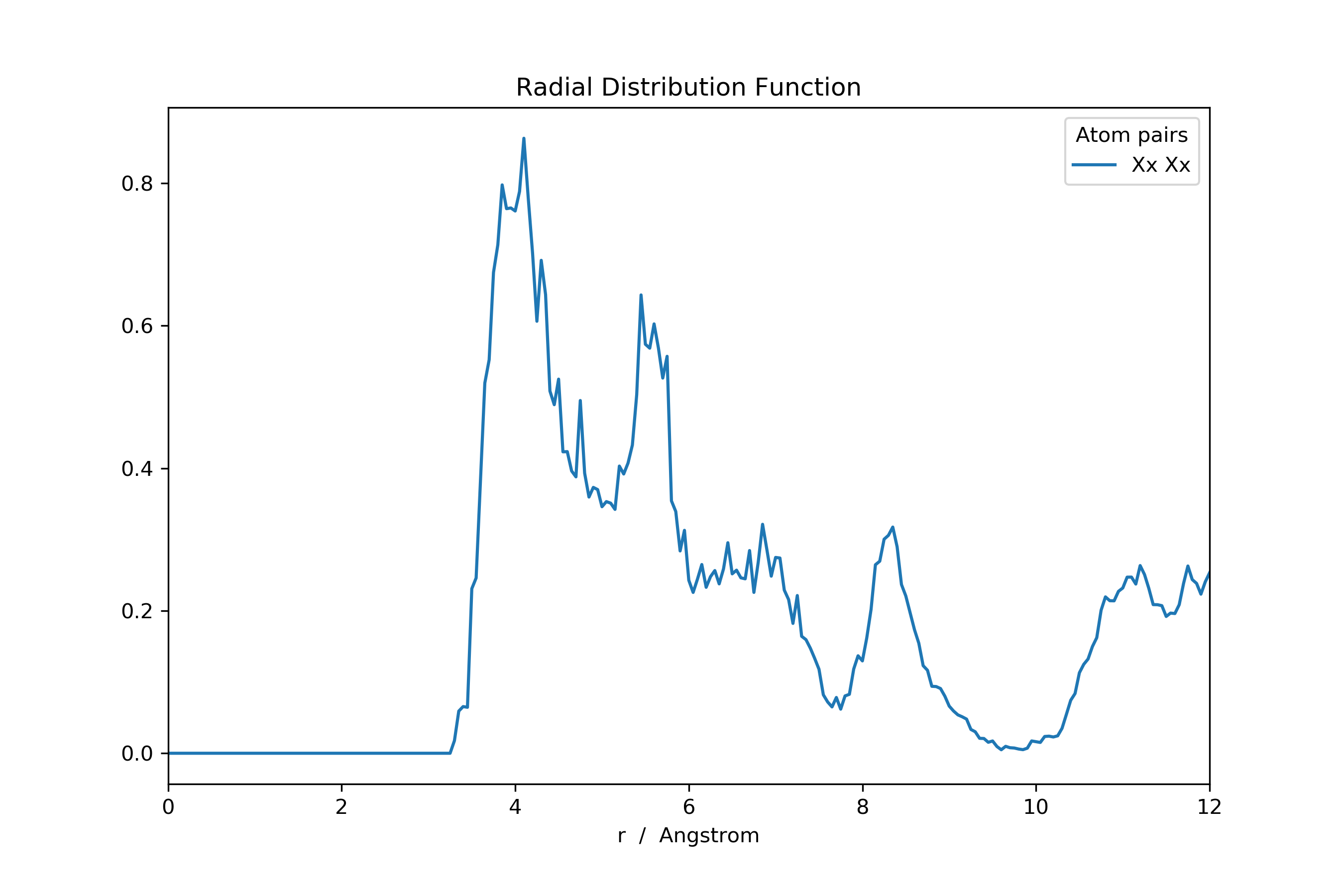Or the ADF.

>>> ...

>>> adf: pd.DataFrame = mol_new.init_rdf(atom_subset=['Xx'], r_max=np.inf)Or the potential of mean force (i.e. Boltzmann-inverted RDF).

>>> ...

>>> from scipy import constants
>>> from scm.plams import Units

>>> RT: float = 298.15 * constants.Boltzmann
>>> kj_to_kcal: float = Units.conversion_ratio('kj/mol', 'kcal/mol')

>>> with np.errstate(divide='ignore'):
>>>     rdf_invert: pd.DataFrame = -RT * np.log(rdf) * kj_to_kcal
>>>     rdf_invert[rdf_invert == np.inf] = np.nan  # Set all infinities to not-a-numberFocus on a specific ligand subset is possible by slicing the new ligand Cartesian coordinate array.

>>> ...

>>> keep_lig = [0, 1, 2, 3]  # Keep these ligands; disgard the rest
>>> lig_centra_subset = lig_centra[:, keep_lig]

# Add dummy atoms to the ligand-center of mass and calculate the RDF
>>> mol_new2: MultiMolecule = mol.add_atoms(lig_centra_subset, symbols='Xx')
>>> rdf: pd.DataFrame = mol_new2.init_rdf(atom_subset=['Xx'])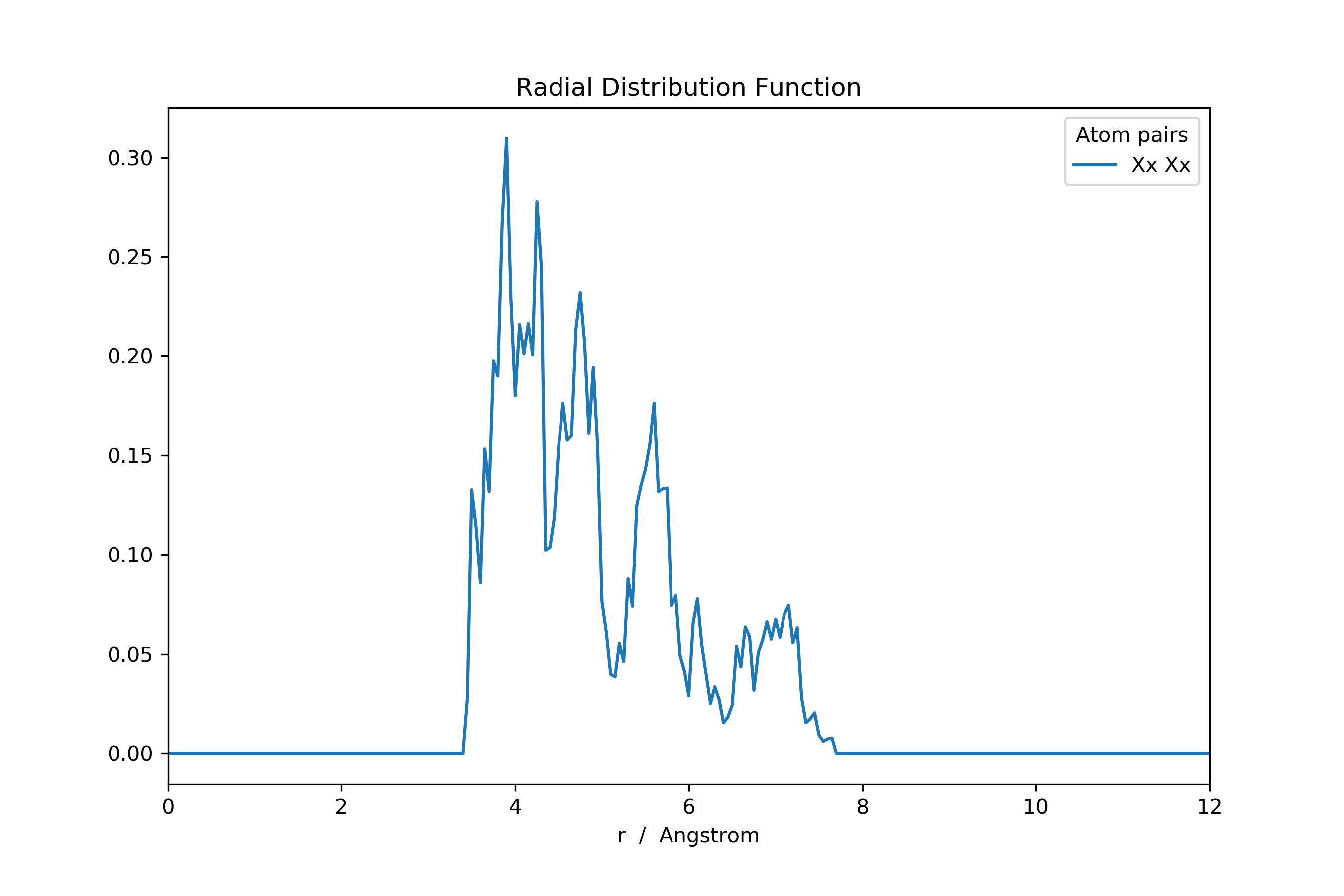Examples

An example for generating a ligand center of mass RDF from a quantum dot with multiple unique ligands. A .psf file will herein be used as starting point.

>>> import numpy as np
>>> from FOX import PSFContainer, MultiMolecule, group_by_values
>>> from FOX.recipes import get_multi_lig_center

>>> mol = MultiMolecule.from_xyz(...)
>>> psf = PSFContainer.read(...)

# Gather the indices of each ligand
>>> idx_dict: dict = group_by_values(enumerate(psf.residue_id, start=1))
>>> del idx_dict  # Delete the core

# Use the .psf segment names as symbols
>>> symbols = [psf.segment_name[i].iloc for i in idx_dict.values()]

# Add dummy atoms to the ligand-center of mass and calculate the RDF
>>> lig_centra: np.ndarray = get_multi_lig_center(mol, idx_dict.values())
>>> mol_new: MultiMolecule = mol.add_atoms(lig_centra, symbols=symbols)
>>> rdf = mol_new.init_rdf(atom_subset=set(symbols))


### Index¶

 get_lig_center(mol, start, step[, stop, …]) Return an array with the (mass-weighted) mean position of each ligands in mol. get_multi_lig_center(mol, idx_iter[, …]) Return an array with the (mass-weighted) mean position of each ligands in mol.

### API¶

FOX.recipes.ligands.get_lig_center(mol, start, step, stop=None, mass_weighted=True)[source]

Return an array with the (mass-weighted) mean position of each ligands in mol.

Parameters: mol (MultiMolecule) – A MultiMolecule instance. start (int) – The atomic index of the first ligand atoms. step (int) – The number of atoms per ligand. stop (int, optional) – Can be used for neglecting any ligands beyond a user-specified atomic index. mass_weighted (bool) – If True, return the mass-weighted mean ligand position rather than its unweighted counterpart. A new array with the ligand’s centra of mass. If mol.shape == (m, n, 3) then, given k new ligands, the to-be returned array’s shape is (m, k, 3). numpy.ndarray
FOX.recipes.ligands.get_multi_lig_center(mol, idx_iter, mass_weighted=True)[source]

Return an array with the (mass-weighted) mean position of each ligands in mol.

Contrary to get_lig_center(), this function can handle molecules with multiple non-unique ligands.

Parameters: mol (MultiMolecule) – A MultiMolecule instance. idx_iter (Iterable [Sequence [int]]) – An iterable consisting of integer sequences. Each integer sequence represents a single ligand (by its atomic indices). mass_weighted (bool) – If True, return the mass-weighted mean ligand position rather than its unweighted counterpart. A new array with the ligand’s centra of mass. If mol.shape == (m, n, 3) then, given k new ligands (aka the length of idx_iter) , the to-be returned array’s shape is (m, k, 3). numpy.ndarray